# MOSFET (Si/SiC) Forum Discussions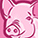Level 1Level 1

# SPICE Model for irhys9a7234cm (250 volt n-ch MOSFET) has curiously low value for the non-linear CGDO which results in much overshoot at the gate when I include parasitic inductance of 6.8 nH on leads

I brought the PSPICE model into LTSPICE and added external stray inductances of 6.8 nH to the leads and found that there was up to 35 volts of overshoot at the gate even with 10 Ohm external gate resistor.

I know that the overshoot is caused by resonance from the inductance and the effective gate capacitance of which CGDO contributes and that critical damping occurs when the gate resistor = 1/2 x sqrt (L_stray/C).  So a low value of capacitance would result in an underdamped response with ringing.

I then looked at the model and found that the CGDO value was only 10 pF.  I looked at a similar  Power MOSFET and it had much higher CGDO, about 68 nF.  I then removed the IRHYS9A7234CM model from my schematic and substituted the other MOSFET model.  I saw no ringing or overshoot with the other model.

My question is :  is the CGDO value correct in the irhys9a7234cm  model?

I pasted the SPICE model for the IRHYS9A7234CM (that exhibits the overshoot) below.

Regards,

Ken

.SUBCKT irhys9a7234cm 1 2 3
**************************************
* Model Generated by MODPEX *
* Contains Proprietary Information *
* Which is The Property of *
* SYMMETRY OR ITS LICENSORS *
*Commercial Use or Resale Restricted *
* by Symmetry License Agreement *
**************************************
* Model generated on Nov 7, 19
* MODEL FORMAT: SPICE3
* Symmetry POWER MOS Model (Version 1.0)
* External Node Designations
* Node 1 -> Drain
* Node 2 -> Gate
* Node 3 -> Source
M1 9 7 8 8 MM L=100u W=100u
.MODEL MM NMOS LEVEL=1 IS=1e-32
+VTO=3.90376 LAMBDA=0.129709 KP=33.9959
+CGSO=1.60177e-05 CGDO=1e-11
RS 8 3 0.0804021
D1 3 1 MD
.MODEL MD D IS=9.9526e-09 RS=0.011175 N=1.5 BV=250
+IBV=0.001 EG=1 XTI=3.09708 TT=1e-07
+CJO=1.10724e-08 VJ=0.5 M=0.9 FC=0.5
RDS 3 1 5e+07
RD 9 1 0.0001
RG 2 7 21.1069
D2 4 5 MD1
* Default values used in MD1:
* RS=0 EG=1.11 XTI=3.0 TT=0
* BV=infinite IBV=1mA
.MODEL MD1 D IS=1e-32 N=50
+CJO=3.56975e-11 VJ=0.5 M=0.9 FC=1e-08
D3 0 5 MD2
* Default values used in MD2:
* EG=1.11 XTI=3.0 TT=0 CJO=0
* BV=infinite IBV=1mA
.MODEL MD2 D IS=1e-10 N=0.4 RS=3e-06
RL 5 10 1
FI2 7 9 VFI2 -1
VFI2 4 0 0
EV16 10 0 9 7 1
CAP 11 10 1.17096e-09
FI1 7 9 VFI1 -1
VFI1 11 6 0
RCAP 6 10 1
D4 0 6 MD3
* Default values used in MD3:
* EG=1.11 XTI=3.0 TT=0 CJO=0
* RS=0 BV=infinite IBV=1mA
.MODEL MD3 D IS=1e-10 N=0.4
.ENDS irhys9a7234cm

1 SolutionModeratorModerator

# Re: SPICE Model for irhys9a7234cm (250 volt n-ch MOSFET) has curiously low value for the non-linear CGDO which results in much overshoot at the gate when I include parasitic inductance of 6.8 nH on le

Hello,

Thank you for sharing the model. I realized in the datasheet it is mentioned Ls+Ld,the total inductance value equals 6.8nH, not individually. We cannot take the lead inductance value as 6.8nH it should be lower than that(for Ls and Ld). Putting any value greater than 6.8nH will worsen the situation and result in an overshoot.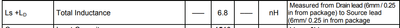Regards,
Anshika

9 RepliesModeratorModerator

# Re: SPICE Model for irhys9a7234cm (250 volt n-ch MOSFET) has curiously low value for the non-linear CGDO which results in much overshoot at the gate when I include parasitic inductance of 6.8 nH on le

Hello Ken,
Thankyou for posting on Infineon Community.
The Infineon spice models have verified models and should result accurately with the specifications provided in the model.
I infer that you want to make your circuit operate at resonant conditions and that is why you are adding external inductance to it. Please let me know if there is something  more to it.

Can you please check without the external inductance or with a small value of it and share the results?

If you stimulate the resonant circuit with a fast positive or negative edge, it will resonate, which includes overshoot and undershoot.  I understand that when you have tried with a high capacitance value , the gate resistor value will be small and it results in no overshoot.

Regards,
AnshikaLevel 1Level 1

# Re: SPICE Model for irhys9a7234cm (250 volt n-ch MOSFET) has curiously low value for the non-linear CGDO which results in much overshoot at the gate when I include parasitic inductance of 6.8 nH on le

I attached a set of slides showing the gate voltage overshoot with 0.001 nH (basically no inductance) in series with each of the three MOSFET leads vs 6.8 nH in series with each of the MOSFET leads (which is the datasheet lead inductance) on all three leads.

In both cases the gate resistance is 2 Ohms and is modeled as an external resistor on the simulation schematic between the MOSFET driver and the MOSFET gate lead.

There is an unexpected overshoot at the gate that pushes the gate voltage above the 20 volt absolute maximum.ModeratorModerator

# Re: SPICE Model for irhys9a7234cm (250 volt n-ch MOSFET) has curiously low value for the non-linear CGDO which results in much overshoot at the gate when I include parasitic inductance of 6.8 nH on le

Hello,
Thank you for sharing the results.

From the graphs shared by you, it is clear that Cgdo value alone does not cause any issue if used without inductance, but overshoots when do.
I am hoping that you have considered both the capacitances Cgso and Cgdo in your simulation model. If not, please do as Cgso has great significance while doing simulation or real-time modelling.

Cgso and Cgdo are overlap capacitance that occur because of some manufacture imperfections or so, they are not junction capacitances as Cgs and Cgd. In the datasheet, the value of input capacitance Ciss is given, can you please use this value and test your circuit: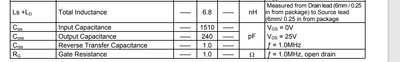If possible, please share your circuit so that I can also test it and answer you in a better way.

Regards,
AnshikaLevel 1Level 1

# Re: SPICE Model for irhys9a7234cm (250 volt n-ch MOSFET) has curiously low value for the non-linear CGDO which results in much overshoot at the gate when I include parasitic inductance of 6.8 nH on le

I don't see Ciss in the SPICE model I am using (pasted here).  Am I missing it?

I know that Ciss is a combination of Cgs and Cgd and that Cgd changes with VDS voltage (because of the Miller Effect), so I'm just wondering how to accurately model Ciss (without, for instance, an if-then statement to account for the various values of Cgd during the various stages of turn-on).

I'll work on getting you a simulation file that you can run.  I may have to get approval to send it to you.

Ken

.SUBCKT irhys9a7234cm 1 2 3
**************************************
* Model Generated by MODPEX *
* Contains Proprietary Information *
* Which is The Property of *
* SYMMETRY OR ITS LICENSORS *
*Commercial Use or Resale Restricted *
* by Symmetry License Agreement *
**************************************
* Model generated on Nov 7, 19
* MODEL FORMAT: SPICE3
* Symmetry POWER MOS Model (Version 1.0)
* External Node Designations
* Node 1 -> Drain
* Node 2 -> Gate
* Node 3 -> Source
M1 9 7 8 8 MM L=100u W=100u
.MODEL MM NMOS LEVEL=1 IS=1e-32
+VTO=3.90376 LAMBDA=0.129709 KP=33.9959
+CGSO=1.60177e-05 CGDO=1e-11
RS 8 3 0.0804021
D1 3 1 MD
.MODEL MD D IS=9.9526e-09 RS=0.011175 N=1.5 BV=250
+IBV=0.001 EG=1 XTI=3.09708 TT=1e-07
+CJO=1.10724e-08 VJ=0.5 M=0.9 FC=0.5
RDS 3 1 5e+07
RD 9 1 0.0001
RG 2 7 21.1069
D2 4 5 MD1
* Default values used in MD1:
* RS=0 EG=1.11 XTI=3.0 TT=0
* BV=infinite IBV=1mA
.MODEL MD1 D IS=1e-32 N=50
+CJO=3.56975e-11 VJ=0.5 M=0.9 FC=1e-08
D3 0 5 MD2
* Default values used in MD2:
* EG=1.11 XTI=3.0 TT=0 CJO=0
* BV=infinite IBV=1mA
.MODEL MD2 D IS=1e-10 N=0.4 RS=3e-06
RL 5 10 1
FI2 7 9 VFI2 -1
VFI2 4 0 0
EV16 10 0 9 7 1
CAP 11 10 1.17096e-09
FI1 7 9 VFI1 -1
VFI1 11 6 0
RCAP 6 10 1
D4 0 6 MD3
* Default values used in MD3:
* EG=1.11 XTI=3.0 TT=0 CJO=0
* RS=0 BV=infinite IBV=1mA
.MODEL MD3 D IS=1e-10 N=0.4
.ENDS irhys9a7234cmModeratorModerator

# Re: SPICE Model for irhys9a7234cm (250 volt n-ch MOSFET) has curiously low value for the non-linear CGDO which results in much overshoot at the gate when I include parasitic inductance of 6.8 nH on le

Hi Ken,

Yes,you are correct,the Ciss is not mentioned in the SPICE model and it would be difficult to analyze the behavior accurately. Have you checked for Cgso value also for the irhys9a7234cm and the other Mosfet that you have used?

I would appreciate if you can share the model.

Regards,
AnshikaLevel 1Level 1

# Re: SPICE Model for irhys9a7234cm (250 volt n-ch MOSFET) has curiously low value for the non-linear CGDO which results in much overshoot at the gate when I include parasitic inductance of 6.8 nH on le

Hi ,

Here is a plot of the gate voltage.  I attached the zipped LTSPICE file.  Note that the gate resistor and board inductance associated with the MOSFET pins are parameters that can be changed and right now the gate resistor is fairly large value at 11 Ohms and the stray inductance is 19.7 nH which is a value consistent with 2 inches of AWG18 wire.Level 1Level 1

# Re: SPICE Model for irhys9a7234cm (250 volt n-ch MOSFET) has curiously low value for the non-linear CGDO which results in much overshoot at the gate when I include parasitic inductance of 6.8 nH on le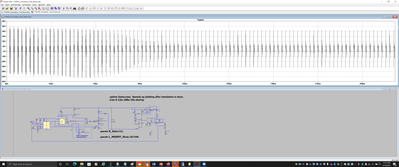ModeratorModerator

# Re: SPICE Model for irhys9a7234cm (250 volt n-ch MOSFET) has curiously low value for the non-linear CGDO which results in much overshoot at the gate when I include parasitic inductance of 6.8 nH on le

Hello,

Thank you for sharing the model. I realized in the datasheet it is mentioned Ls+Ld,the total inductance value equals 6.8nH, not individually. We cannot take the lead inductance value as 6.8nH it should be lower than that(for Ls and Ld). Putting any value greater than 6.8nH will worsen the situation and result in an overshoot.Regards,
Anshika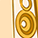Level 2Level 2

# Re: SPICE Model for irhys9a7234cm (250 volt n-ch MOSFET) has curiously low value for the non-linear CGDO which results in much overshoot at the gate when I include parasitic inductance of 6.8 nH on le